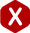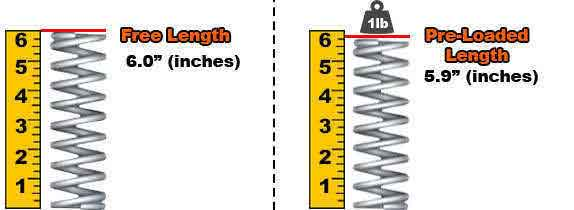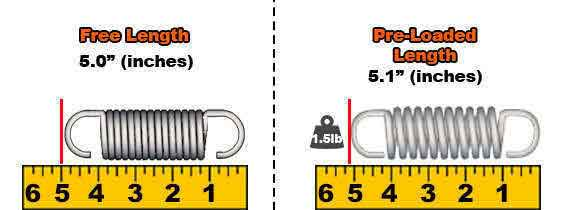<#### Definition: The calculation of a spring’s working loads when it is in a pre-loaded state.

Pre-load is very useful when installing your coil spring into your mechanism or application. Pre-load can help you hold your spring in place due to the tension built up in between the components of your mechanism and the spring itself. Its coils will compress or extend slightly therefore building up energy or force which the spring exerts in order to return to its original position.

Pre-load is considered you load 1 (L1) on our spring calculator because it will support a load and therefore, travel an amount of distance corresponding to the spring’s rate or constant. If you are uncertain of how much pre-load is being applied to your spring, you may input either the load being applied or the distance being traveled and the other value will automatically be calculated once you click on calculate. The examples provided below will show what pre-load looks like for a compression and an extension spring. They will also show you how to calculate the amount of pre-load placed on your spring.

#### Example A.) Compression Spring Pre-Load

The calculation of a compression spring’s pre-load is the easiest to calculate out of the three types of common coil springs (compression springs,extension springs, and torsion springs). This value is simply calculated as a regular load as shown in the formulas below. As you can see in the diagram exposed, your spring’s pre-load is a small amount of load applied only to minimally compress the spring for it to release energy and therefore have some kind of grip between the components working around your spring. This will help your spring to stay in place.

This spring has a 6” (inch) free length, a spring rate of 10 lbf/in (pounds of force per inch), and travels 0.1” (inches) under pre-load. So, how much pre-load is being applied to this compression spring?

#### Formula

This spring has a 6” (inch) free length, a spring rate of 10 lbf/in (pounds of force per inch), and travels 0.1” (inches) under pre-load. So, how much pre-load is being applied to this compression spring?

pL = k(T)
• k = Rate
• T = Distance Traveled
pL = 10 (0.1)
pL = 1 lbf (pounds of force)

#### Diagram#### Formula

This spring has a 5” length inside hooks, a spring rate of 5 lbf., an initial tension of 1 lbf. and it travels 0.1” under pre-load. So, how much preload is sustaining this extension spring?

pL = k(T) + iT